## Energy Transfer Diagrams

Most of the machines or devices we use transfer energy from one form to another. Input energy is taken in by the device in one form and transformed to output energy in another form.

An energy transfer diagram or a Sankey diagram is used to show the transfer of energy across a process or a device. It is a flow diagram in which the widths of the arrows show the relative amounts of each type of energy.

An energy transfer diagram for a power station along with a Sankey diagram is shown below: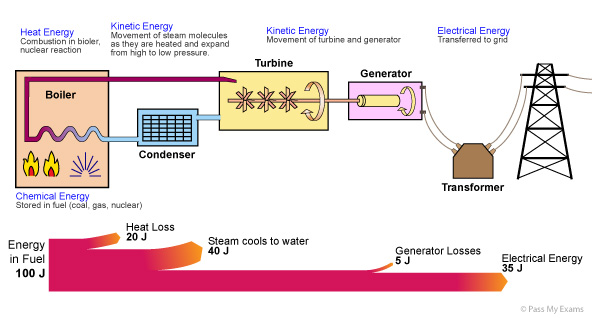## Efficiency

The efficiency of a device is calculated using the following formula: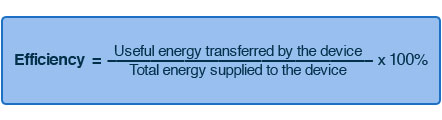### Light Bulb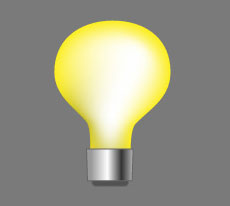Energy Transfer Diagram:

Electrical Energy → Heat and Light Energy

Sankey Diagram: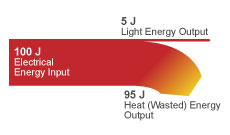Efficiency:

Efficiency = 5/100 x 100
Efficiency = 5%

Explanation:

Energy cannot be created nor destroyed. It can only be transformed from one form to another. Thus the 100J of electrical energy is transformed to 5 J of light energy and 95J of heat energy. In the case of the light bulb the 95J of energy transferred as heat is wasted energy as it is not useful because the purpose of the device is to produce light.

An ordinary light bulb works on the principle of a thin wire (filament) being heated by the resistance to the electrical current. At a temperature of about 1100°C it glows with a bright white light. As the electrical energy is required in heating the wire hence this is why most of the energy is given off as heat. Lamps which give of light when hot are called incandescent.

### Energy Saving Light Bulb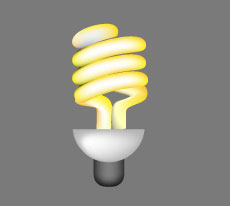Energy Transfer Diagram:

Electrical Energy → Heat and Light Energy

Sankey Diagram: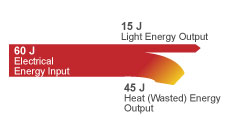Efficiency

Efficiency = 15/60 x 100
Efficiency = 25%

Explanation:

Energy saving light bulbs work on the principle of fluorescence. Here the electrical energy is supplied to electrodes which generate fast moving electrons that pass through a tube containing mercury gas. On collision with the mercury atoms ultraviolet light is produced which then collides with the phosphor atoms coated around the tube converting the ultraviolet to visible light.

Here a greater proportion of the electrical energy is converted to useful light, thus the energy saving light bulb is a more efficient device.

Energy cannot be created nor destroyed. It can only be transformed from one form to another (the law of conservation of energy).

When energy is transformed or transferred only part of it can be usefully transformed or transferred. The energy which is not usefully transformed or transferred is referred to as wasted energy.

Both the useful energy and the wasted energy which is transformed or transferred are eventually transferred to their surroundings which become warmer. As the energy spreads out it becomes more difficult to use for further energy transformations.

The greater the percentage of the energy that can be usefully transformed by a device the higher its efficiency.